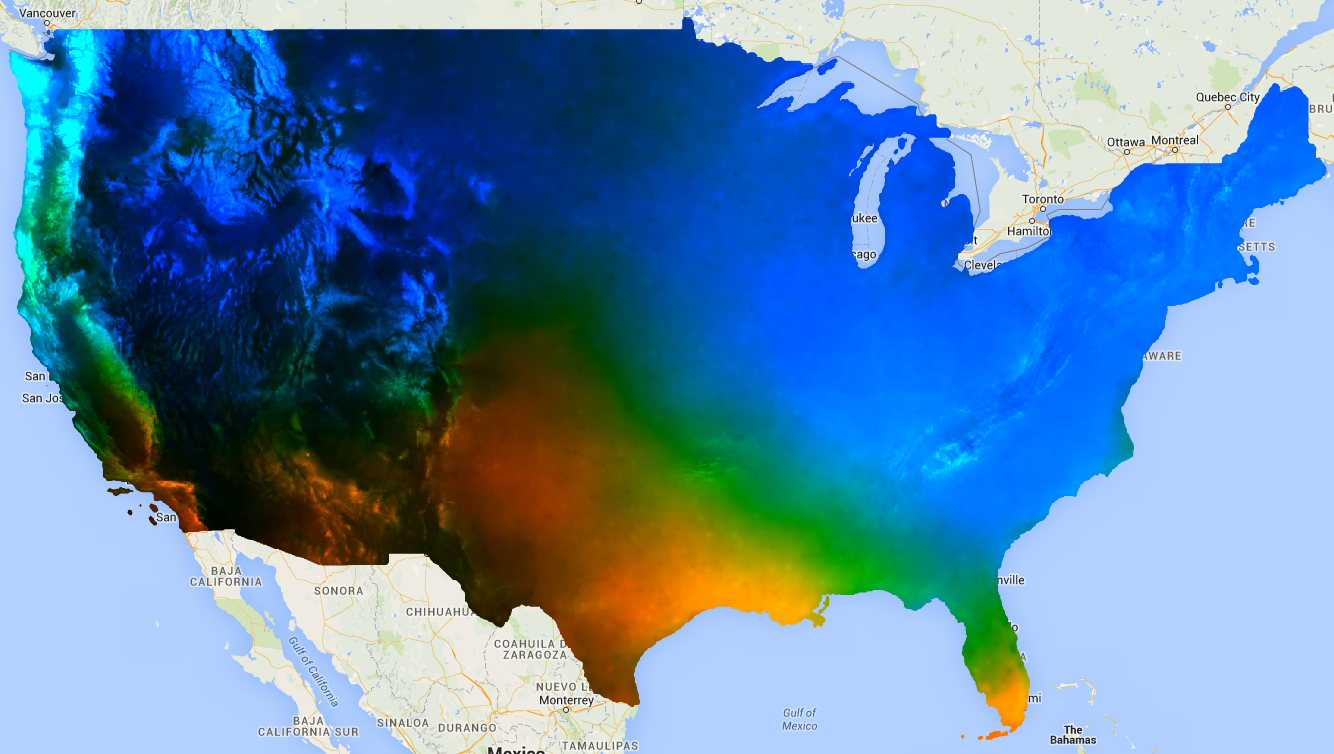# Linear Regression

Earth Engine contains a variety of methods for performing linear regression using reducers. The simplest linear regression reducer is `ee.Reducer.linearFit()` which computes the least squares estimate of a linear function of one variable with a constant term. The data should be set up as a two-band input image, where the first band is the independent variable and the second band is the dependent variable. The following example shows estimation of the linear trend of future precipitation (after 2006 in the NEX data) projected by climate models. The dependent variable is projected precipitation and the independent variable is time, added prior to calling `linearFit()`:

```// This function adds a time band to the image.
var createTimeBand = function(image) {
// Scale milliseconds by a large constant to avoid very small slopes
// in the linear regression output.
};

// Load the input image collection: projected climate data.
var collection = ee.ImageCollection('NASA/NEX-DCP30_ENSEMBLE_STATS')
.filter(ee.Filter.eq('scenario', 'rcp85'))
.filterDate(ee.Date('2006-01-01'), ee.Date('2050-01-01'))
// Map the time band function over the collection.
.map(createTimeBand);

// Reduce the collection with the linear fit reducer.
// Independent variable are followed by dependent variables.
var linearFit = collection.select(['system:time_start', 'pr_mean'])
.reduce(ee.Reducer.linearFit());

// Display the results.
Map.setCenter(-100.11, 40.38, 5);
{min: 0, max: [-0.9, 8e-5, 1], bands: ['scale', 'offset', 'scale']}, 'fit');
```

Observe that the output contains two bands, the `‘offset’` (intercept) and the `‘scale’` ('scale' in this context refers to the slope of the line and is not to be confused with the scale parameter input to many reducers, which is the spatial scale). The result, with areas of increasing trend in blue, decreasing trend in red and no trend in green should look something like Figure 1.Figure 1. The output of linearFit() applied to projected precipitation. Areas projected to be increased precipitation are shown in blue and decreased precipitation in red.

For a more flexible approach to linear modelling, use one of the linear regression reducers which allow for a variable number of independent and dependent variables. Specifically, `ee.Reducer.linearRegression()` implements ordinary least squares regression (OLS). Alternatively, `robustLinearRegression()` uses a cost function based on regression residuals to iteratively de-weight outliers in the data (O’Leary 1990).

For example, suppose there are two dependent variables: precipitation and maximum temperature, and two independent variables: a constant and time. The collection is identical to the previous example, but the constant band must be manually added prior to the reduction. The first two bands of the input are the ‘X’ (independent) variables and the next two bands are the ‘Y’ (dependent) variables. In this example, first get the regression coefficients, then flatten the array image to extract the bands of interest:

```// This function adds a time band to the image.
var createTimeBand = function(image) {
// Scale milliseconds by a large constant.
};

// This function adds a constant band to the image.
var createConstantBand = function(image) {
};

// Load the input image collection: projected climate data.
var collection = ee.ImageCollection('NASA/NEX-DCP30_ENSEMBLE_STATS')
.filterDate(ee.Date('2006-01-01'), ee.Date('2099-01-01'))
.filter(ee.Filter.eq('scenario', 'rcp85'))
// Map the functions over the collection, to get constant and time bands.
.map(createTimeBand)
.map(createConstantBand)
// Select the predictors and the responses.
.select(['constant', 'system:time_start', 'pr_mean', 'tasmax_mean']);

// Compute ordinary least squares regression coefficients.
var linearRegression = collection.reduce(
ee.Reducer.linearRegression({
numX: 2,
numY: 2
}));

// Compute robust linear regression coefficients.
var robustLinearRegression = collection.reduce(
ee.Reducer.robustLinearRegression({
numX: 2,
numY: 2
}));

// The results are array images that must be flattened for display.
// These lists label the information along each axis of the arrays.
var bandNames = [['constant', 'time'], // 0-axis variation.
['precip', 'temp']]; // 1-axis variation.

// Flatten the array images to get multi-band images according to the labels.
var lrImage = linearRegression.select(['coefficients']).arrayFlatten(bandNames);
var rlrImage = robustLinearRegression.select(['coefficients']).arrayFlatten(bandNames);

// Display the OLS results.
Map.setCenter(-100.11, 40.38, 5);
{min: 0, max: [-0.9, 8e-5, 1], bands: ['time_precip', 'constant_precip', 'time_precip']}, 'OLS');

// Compare the results at a specific point:
print('OLS estimates:', lrImage.reduceRegion({
reducer: ee.Reducer.first(),
geometry: ee.Geometry.Point([-96.0, 41.0]),
scale: 1000
}));

print('Robust estimates:', rlrImage.reduceRegion({
reducer: ee.Reducer.first(),
geometry: ee.Geometry.Point([-96.0, 41.0]),
scale: 1000
}));
```

Inspect the results to discover that `linearRegression()` output is equivalent to the coefficients estimated by the `linearFit()` reducer, though the `linearRegression()` output also has coefficients for the other dependent variable, `tasmax_mean`. Robust linear regression coefficients are different from the OLS estimates. The example compares the coefficients from the different regression methods at a specific point.

Текущей странице